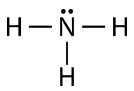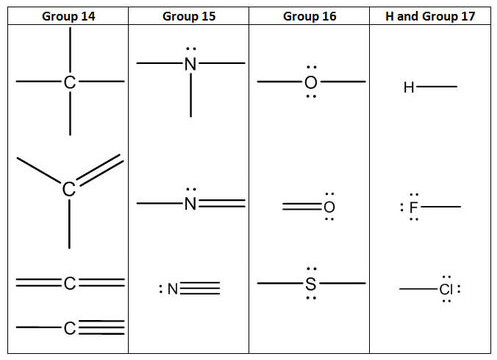# 4.1: Lewis Electron Dot Structures

•• Contributed by Allison Soult
• Senior Lecturer (Chemistry) at University of Kentucky

Learning Outcomes

• Determine Lewis structure of a compound.

Ionic substances are completely held together by ionic bonds. The full charges of the ions (for example, $$\ce{Na^+}$$ and $$\ce{Cl^-}$$ in sodium chloride) cause electrostatic interactions that result in a stable crystal lattice. Ionic compounds exist as extended, orderly arrangements of ions. This is quite different from the structure of molecular substances, which take the form of collections of individual molecules.

The electrons that form a covalent bond are not fully possessed by a single atom (as the electrons in an ion would be) but are shared between the two atoms involved in the bond. The concept of the covalent bond was first proposed in 1916 by the American chemist G. N. Lewis (1875 - 1946), who suggested that sharing electrons was one way that atoms could attain a complete octet of valence electrons. This idea was expanded upon by Linus Pauling (1901 - 1994), who eventually won the Nobel Prize in Chemistry in 1954 for his work on chemical bonding.

## Single Covalent Bonds

The simplest covalent bond is formed between two hydrogen atoms. Each hydrogen atom has a single electron, and each needs two electrons for a full outer shell. The hydrogen molecule, $$\ce{H_2}$$, consists of two hydrogen atoms sharing their two valence electrons. Hydrogen can also form covalent bonds with other atoms. For example, hydrogen and chlorine each need one more electron to achieve a noble gas configuration. By sharing valence electrons (each atom donates one), the stable $$\ce{HCl}$$ molecule is formed.

We will use a simplified representation of covalent bonds known as Lewis structures. These drawings are also know by various other names, including Lewis dot structures or electron dot structures. Each dot in the structure represents one valence electron in the compound. For example, $$\ce{H_2}$$ could be drawn as $$\ce{H} : \ce{H}$$. Each dot represents one valence electron, and the fact that they are placed between the two atoms means that they are being shared bas a covalent bond. For larger molecules, it can become cumbersome to draw out all of the valence electrons, so a bonding pair of electrons can also be drawn as a straight line. Thus, $$\ce{H_2}$$ can also be represented as $$\ce{H-H}$$.

If we wanted to show the Lewis structure of $$\ce{HCl}$$, we would draw the following:We can see that the covalent bond consists of two electrons between the $$\ce{H}$$ and the $$\ce{Cl}$$. The $$\ce{H}$$ has a full outer shell of two electrons and the chlorine has a full outer shell of eight electrons. Covalent bonds with other halogens can be written the same way.

Similar types of Lewis structures can be written for other molecules that form covalent bonds. Many compounds that contain $$\ce{O}$$, $$\ce{N}$$, $$\ce{C}$$, $$\ce{S}$$, and $$\ce{P}$$ are held together by covalent bonds. The number of covalent bonds an atom will form can generally be predicted by the number of electrons an atom requires to fill its valence shell. For example, oxygen has 6 electrons in its outer shell and needs two more to fill this shell, so it will only form two covalent bonds with other atoms. If we look at the water molecule $$\left( \ce{H_2O} \right)$$ (see figure below), we see that the oxygen atom makes two total bonds (one with each hydrogen atom).Figure $$\PageIndex{1}$$: Electron sharing in a water molecule.

As you can see, there are two pairs of electrons not involved in covalent bonding. These unbonded pairs of electrons are known as lone pairs and contribute to the overall shape of the molecule. Similarly, nitrogen needs three electrons to complete its valence shell, so it tends to make three covalent bonds, with one lone pair of non-bonding electrons left over.Again, each of the lines stands for a pair of bonding electrons (a single bond), and the lone pair of nitrogen is drawn as two dots.

## Double and Triple Bonds

So far we have considered only single bonds, formed by the sharing of one electron from each atom. Many molecules contain double bonds, in which each atom shares two electrons, or triple bonds, in which each atom shares three electrons. These are represented by drawing two or three lines between the bonded atoms. For example, a carbon-carbon double bond can be written as $$\ce{C} :: \ce{C}$$ or $$\ce{C=C}$$. A carbon-carbon triple bond is shown as $$\ce{C ::: C}$$ or with three lines between the two carbon atoms, as seen in the structure of an organic molecule called acetylene (shown in the figure below). Just as $$\ce{N}$$ wants to form 3 bonds, other elements tend to form the same number of bonds in different compounds.Figure $$\PageIndex{2}$$: Acetylene molecule.

We will build Lewis structures that satisfy the octet rule to determine how atoms are attached to one another using the components shown in the table below to create structures that follow the octet rule.

Table $$\PageIndex{1}$$: Components to Build Lewis Structures